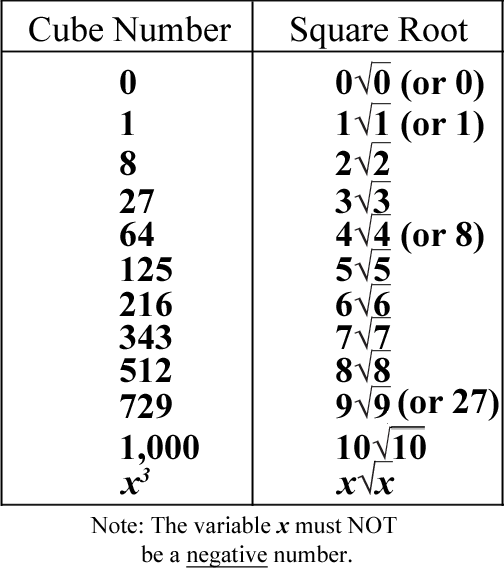# The Square Roots of Cubes

## Behold the pattern of the square roots of cubes! (Of course, the cubes are integers!)### According to the pattern, the square root of a cube of a number x is equal to x multiplied by the square root of x! (If x is a positive number or zero.)

Bonus Info: 8 × its square root is the same as 16 × the square root of 2!